# compare the OpenPIV Python with PIVLab¶

Analysis of the Karman images final int area 6 pixels and 50% overlap, vector validation is allowed, but no smoothing after the last correlation. Only the circle in the middle must be masked, not the shadows. Then we can compare the vorticity maps (color bar scale of uncalibrated data -0.3 1/frame until +0.3 1/frame, color map preferably "parula", but "jet" is also ok). That might give an idea about the "quality"...?
In :
%reload_ext watermark
%watermark -v -m -p numpy,openpiv

Python implementation: CPython
Python version       : 3.8.5
IPython version      : 7.19.0

numpy  : 1.19.2
openpiv: 0.23.5

Compiler    : GCC 7.3.0
OS          : Linux
Release     : 5.4.0-70-generic
Machine     : x86_64
Processor   : x86_64
CPU cores   : 8
Architecture: 64bit


In :
from openpiv import windef
from openpiv import tools, scaling, validation, filters, preprocess
from openpiv.pyprocess import extended_search_area_piv, get_field_shape, get_coordinates
from openpiv import smoothn
from openpiv.preprocess import mask_coordinates

import numpy as np
import os
from time import time
import warnings

import matplotlib.pyplot as plt
%matplotlib inline

import matplotlib
matplotlib.rcParams['figure.figsize'] = (10.0, 8.0)

In :
settings = windef.Settings()

# 'Data related settings'
# Folder with the images to process
settings.filepath_images = '../test9/'
# Folder for the outputs
settings.save_path = '.'
# Root name of the output Folder for Result Files
settings.save_folder_suffix = ''
# Format and Image Sequence
settings.frame_pattern_a = 'karman_16Hz_000_A.jpg'
settings.frame_pattern_b = 'karman_16Hz_000_B.jpg'

'Region of interest'
# (50,300,50,300) #Region of interest: (xmin,xmax,ymin,ymax) or 'full' for full image
settings.ROI = 'full'
# settings.ROI = (200,400,600,850)

settings.deformation_method = 'symmetric' # or 'second image'

settings.num_iterations = 4  # select the number of PIV passes

# add the interrogation window size for each pass.
# For the moment, it should be a power of 2
settings.windowsizes=(32, 16, 8, 6)
settings.overlap=(16, 8, 4, 3)

# settings.windowsizes = (128, 64, 32, 16, 8) # if longer than n iteration the rest is ignored
# The overlap of the interroagtion window for each pass.
# settings.overlap = (64, 32, 16, 8, 4) # This is 50% overlap

# Has to be a value with base two. In general window size/2 is a good choice.
# methode used for subpixel interpolation: 'gaussian','centroid','parabolic'
settings.subpixel_method = 'gaussian'

# order of the image interpolation for the window deformation
settings.interpolation_order = 1
settings.scaling_factor = 1  # scaling factor pixel/meter
settings.dt = 1  # time between to frames (in seconds)
'Signal to noise ratio options (only for the last pass)'
# It is possible to decide if the S/N should be computed (for the last pass) or not
# settings.extract_sig2noise = True  # 'True' or 'False' (only for the last pass)
# method used to calculate the signal to noise ratio 'peak2peak' or 'peak2mean'
settings.sig2noise_method = 'peak2peak'
# select the width of the masked to masked out pixels next to the main peak
settings.sig2noise_mask = 2
# If extract_sig2noise==False the values in the signal to noise ratio
# output column are set to NaN

# only effecting the first pass of the interrogation the following passes
# in the multipass will be validated

'Output options'
# Select if you want to save the plotted vectorfield: True or False
settings.save_plot = False
# Choose wether you want to see the vectorfield or not :True or False
settings.show_plot = True
settings.scale_plot = 200  # select a value to scale the quiver plot of the vectorfield
# run the script with the given settings

# 'Processing Parameters'
settings.correlation_method='linear'  # 'circular' or 'linear'
settings.normalized_correlation = True

# 'vector validation options'
# choose if you want to do validation of the first pass: True or False
settings.validation_first_pass = True

settings.filter_method = 'localmean'
# maximum iterations performed to replace the outliers
settings.max_filter_iteration = 10
settings.filter_kernel_size = 3  # kernel size for the localmean method

settings.replace_vectors = True

settings.MinMax_U_disp = (-5, 5)
settings.MinMax_V_disp = (-5, 5)

# The second filter is based on the global STD threshold
settings.std_threshold = 3  # threshold of the std validation

# The third filter is the median test (not normalized at the moment)
settings.median_threshold = 3  # threshold of the median validation
# On the last iteration, an additional validation can be done based on the S/N.
settings.median_size=1 #defines the size of the local median, it'll be 3 x 3

settings.dynamic_masking_method = 'intensity'
settings.dynamic_masking_threshold = 0.1
settings.dynamic_masking_filter_size = 21

In :
vars(settings)

Out:
{'filepath_images': '../test9/',
'save_path': '.',
'save_folder_suffix': '',
'frame_pattern_a': 'karman_16Hz_000_A.jpg',
'frame_pattern_b': 'karman_16Hz_000_B.jpg',
'ROI': 'full',
'dynamic_masking_method': 'intensity',
'dynamic_masking_threshold': 0.1,
'dynamic_masking_filter_size': 21,
'correlation_method': 'linear',
'normalized_correlation': True,
'windowsizes': (32, 16, 8, 6),
'overlap': (16, 8, 4, 3),
'num_iterations': 4,
'subpixel_method': 'gaussian',
'deformation_method': 'symmetric',
'interpolation_order': 1,
'scaling_factor': 1,
'dt': 1,
'sig2noise_method': 'peak2peak',
'sig2noise_mask': 2,
'validation_first_pass': True,
'MinMax_U_disp': (-5, 5),
'MinMax_V_disp': (-5, 5),
'std_threshold': 3,
'median_threshold': 3,
'median_size': 1,
'sig2noise_threshold': 1.0,
'sig2noise_validate': True,
'replace_vectors': True,
'smoothn': True,
'smoothn_p': 0.05,
'filter_method': 'localmean',
'max_filter_iteration': 10,
'filter_kernel_size': 3,
'save_plot': False,
'show_plot': True,
'scale_plot': 200,
'image_mask': False,
'show_all_plots': False,
'invert': False,
'_FrozenClass__isfrozen': True}

## Read and crop the images¶

In :
file_a = settings.frame_pattern_a
file_b = settings.frame_pattern_b

# " read images into numpy arrays"
frame_a = tools.imread(os.path.join(settings.filepath_images, file_a))
frame_b = tools.imread(os.path.join(settings.filepath_images, file_b))

# " crop to ROI"
if settings.ROI == "full":
pass
else:
frame_a = frame_a[
settings.ROI:settings.ROI,
settings.ROI:settings.ROI
]
frame_b = frame_b[
settings.ROI:settings.ROI,
settings.ROI:settings.ROI
]


## Show the images¶

In :
plt.imshow(frame_a,cmap=plt.cm.gray)

Out:
<matplotlib.image.AxesImage at 0x7f60946a6760>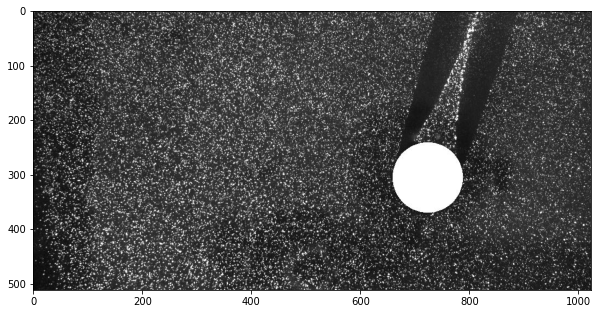## Image masking¶

In :
# 'Image preprocessing'
# 'None' for no masking, 'edges' for edges masking, 'intensity' for intensity masking
# WARNING: This part is under development so better not to use MASKS

if settings.dynamic_masking_method == "edge" or "intensity":
frame_a, image_mask_a = preprocess.dynamic_masking(
frame_a,
method=settings.dynamic_masking_method,
filter_size=settings.dynamic_masking_filter_size,
threshold=settings.dynamic_masking_threshold,
)
frame_b, image_mask_b = preprocess.dynamic_masking(
frame_b,
method=settings.dynamic_masking_method,
filter_size=settings.dynamic_masking_filter_size,
threshold=settings.dynamic_masking_threshold,
)

fig,ax = plt.subplots(1,2)
ax.imshow(frame_a)
ax.imshow(frame_b)

Out:
<matplotlib.image.AxesImage at 0x7f6094498700>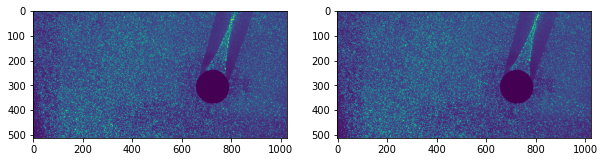In :
# let's combine the two masks if the body is slightly moving
image_mask = np.logical_and(image_mask_a, image_mask_b)
plt.imshow(image_mask)

Out:
<matplotlib.image.AxesImage at 0x7f6094564fd0>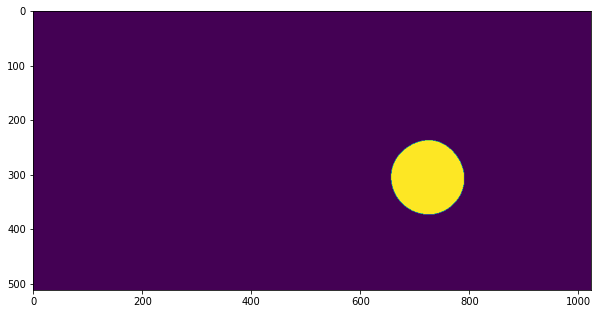## Exract coordinates of the mask as a list of coordinates of a polygon¶

In :
mask_coords = mask_coordinates(image_mask)


## Run the first pass¶

We use typically the most robust approach: linear correlation (with zero padding) and normalized correlation function (0..1)

In :
# In order to convert the image mask to the data mask in x,y
# coordinates, we have to either run first pass or
# use get_coordinates
# Since we do not know how to use the image_mask in the
# first pass with the vectorized correlations, i.e. how to
# save some computational time by skipping the interrogation
# windows within the image mask, we just run the first pass

# "first pass"
x, y, u, v, sig2noise_ratio = windef.first_pass(
frame_a,
frame_b,
settings
)

# store for the comparison of the following steps
u0 = u.copy()
v0 = v.copy()

def status_message(u):
print(f"{np.isnan(u).sum()/u.size*100:.2f}% invalid vectors out of {u.size} vectors")

status_message(u)

1.43% invalid vectors out of 1953 vectors

In :
# Now we can convert the image mask to the data mask in x,y coordinates

from skimage.measure import points_in_poly

# mark those points on the grid of PIV inside the mask
xymask = points_in_poly(np.c_[y.flatten(),x.flatten()],mask_coords)

plt.imshow(~image_mask,cmap=plt.cm.gray)
plt.plot(x.flat[xymask],y.flat[xymask],'x')

Out:
[<matplotlib.lines.Line2D at 0x7f60944f39d0>]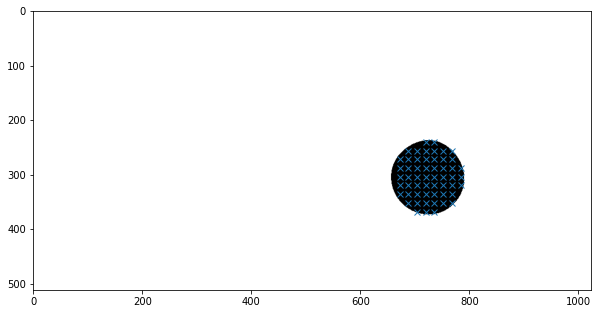In :
# mask the velocity maps for the future use in validation
tmp = np.zeros_like(x,dtype=bool)
tmp.flat[xymask] = True

u = np.ma.masked_array(u, mask = tmp)
v = np.ma.masked_array(v, mask = tmp)

In :
# we need to remove those values for the display
def quick_quiver():
""" u,v expected to have a mask """
plt.quiver(x,y,u,-v,sig2noise_ratio, scale=50,color='b')
plt.gca().invert_yaxis()
plt.gca().set_aspect(1)
plt.plot(x.flat[xymask],y.flat[xymask],'rx')
plt.colorbar(orientation='horizontal')

In :
quick_quiver()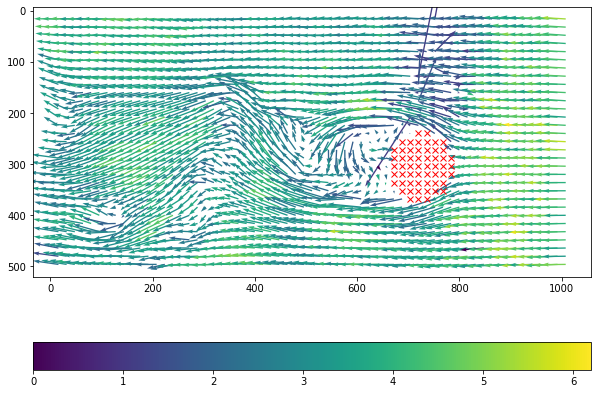In :
# see the distribution of the signal to noise ratio
tmp = sig2noise_ratio.copy()
tmp[tmp>10] = 10  # there are some extra high values 1e7 ...
plt.imshow(tmp)
plt.colorbar(orientation='horizontal')

Out:
<matplotlib.colorbar.Colorbar at 0x7f60903312b0>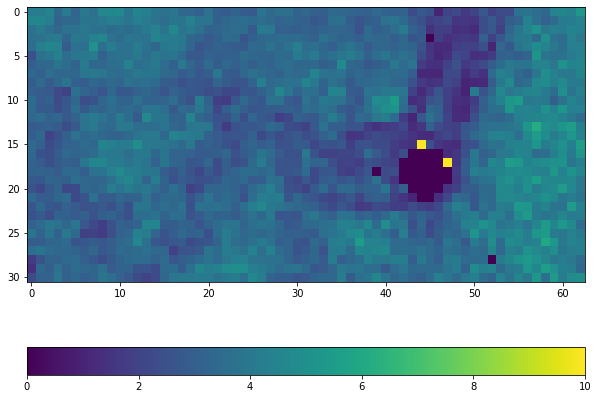In :
plt.hist(tmp.flatten());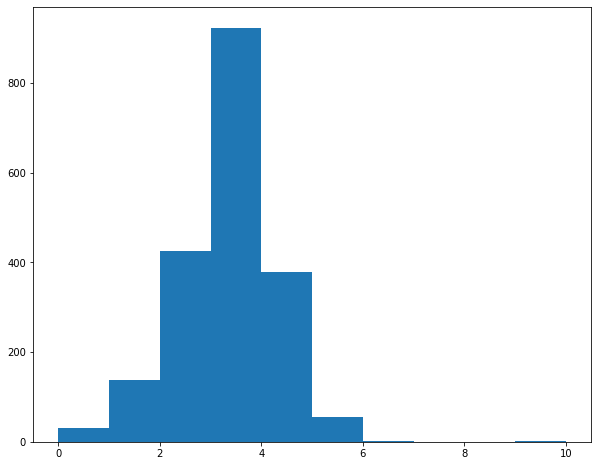In :
# let's consider 5% of signoise ratio problems.
sig2noise_threshold = np.percentile(sig2noise_ratio,(2.5))
print(f"S2N threshold is estimated as {sig2noise_threshold:.3f}")

settings.sig2noise_threshold = 1.2

S2N threshold is estimated as 1.247

In :
u, v, mask_s2n = validation.sig2noise_val(
u, v, sig2noise_ratio,
threshold=settings.sig2noise_threshold
)

status_message(u)

2.30% invalid vectors out of 1953 vectors

In :
plt.figure()
plt.quiver(x,y,u,-v,sig2noise_ratio)
plt.quiver(x[mask_s2n],y[mask_s2n],u0[mask_s2n],-v0[mask_s2n],color='r')
plt.gca().invert_yaxis()
plt.gca().set_aspect(1.)
plt.colorbar(orientation='horizontal')

Out:
<matplotlib.colorbar.Colorbar at 0x7f60900a1250>